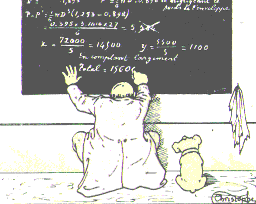# Month: May 2011

Suite du billet du 8 mars 2011. Je reviens à peine d’une semaine de congrès dans les Alpes, à l’École de Physique des Houches, organisée par Catherine Donati-Martin, Sandrine Péché, et Grégory Schehr. Le site est magnifique, face au mont blanc. L’ambiance était excellente. Ces événements scientifiques collectifs me font toujours prendre conscience de l’importance du savoir oral et informel. Ce type de savoir, qui n’a pas vraiment sa place dans les écrits académiques (articles et livres), joue pourtant un rôle considérable dans la formation des esprits et la diffusion des idées. Un blog scientifique permet la publication et la diffusion de ce type de savoir de manière simple et efficace.Let ${X}$ be a ${n\times n}$ random matrix. The circular law theorem states that if ${X}$ has independent and identically distributed entries of variance ${1/n}$ then with probability one, for every bounded continuous ${f:\mathbb{C}\rightarrow\mathbb{R}}$,

$\lim_{n\rightarrow\infty} \frac{1}{n}\sum_{k=1}^nf(\lambda_k(X)) =\frac{1}{\pi}\int_{|z|\leq1}\!f(z)dxdy.$

In other words, the counting probability measure of the eigenvalues of ${X}$ tends weakly to the unifom probability measure on the unit disk ${\{z\in\mathbb{C}:|z|\leq1\}}$ when the dimension ${n}$ goes to infinity. In particular, the counting probability measure of the real part (or the imaginary part) of the eigenvalues of ${X}$ tend to the semicircle law. This theorem, obtained by Tao and Vu in 2009, is actually the final step of a long sequence of partial results due to Mehta, Girko, Silverstein, Bai, Edelman, Goetze and Tikhomirov, Pan and Zhou. The nicest proof is based on logarithmic potential and Hermitization. On the other hand, the complex Gaussian case allows a remarkable direct approach. Note the following stabilization of the second moment which comes from the i.i.d. entries with variance ${1/n}$ (first inequality is due to Weyl, and the last equality is the law of large numbers):

$\frac{1}{n}\sum_{k=1}^n|\lambda_k(X)|^2 \leq \frac{1}{n}\sum_{k=1}^n\lambda_k(XX^*) =\frac{1}{n}\mathrm{Tr}(XX^*) =\frac{1}{n}\sum_{a,b=1}^n|X_{ab}|^2 =O(1).$

Spectral radius. Suppose that ${X}$ has i.i.d. entries with variance ${1/n}$. It is known that with probability one, the spectral radius ${\max_{1\leq k\leq n}|\lambda_k(X)|}$ tends to ${1}$ as ${n}$ goes to infinity. Moreover, it is known that in the complex Gaussian case, the asymptotic fluctuations in this convergence are given by a Gumbel law (and not a Tracy-Widom law). It is natural to conjecture that this Gumbel fluctuation is universal and holds beyond the complex Gaussian case.

Dependent entries. The simplest model with dependence is maybe given by Markov matrices. In the same spirit, it was proved recently by Adamczak that if ${X}$ has i.i.d. rows following an isotropic log-concave density then the circular law holds (apparently to appear in Electronic Journal of Probability, not submitted on arXiv unfortunately). This allows some dependence among the entries of each row, but avoids discrete laws. Intuitively, it is natural to conjecture that the circular is still valid if the law of ${X}$ is invariant by a rich enough set of transformations. For instance, one may conjecture that the circular law holds for random doubly stochastic matrices (Birkhoff polytope equipped with the uniform law). In the same spirit, and more generally, one can conjecture that the circular law holds (up to scaling of course) if the law of ${X}$ is log-concave.

I will give a talk on this subject in École de Physique des Houches (May 24, 2011), and a mini-course in the France-China Summer School (July 10-30, 2011).

Last Updated on 2011-09-18

Syntax · Style · .